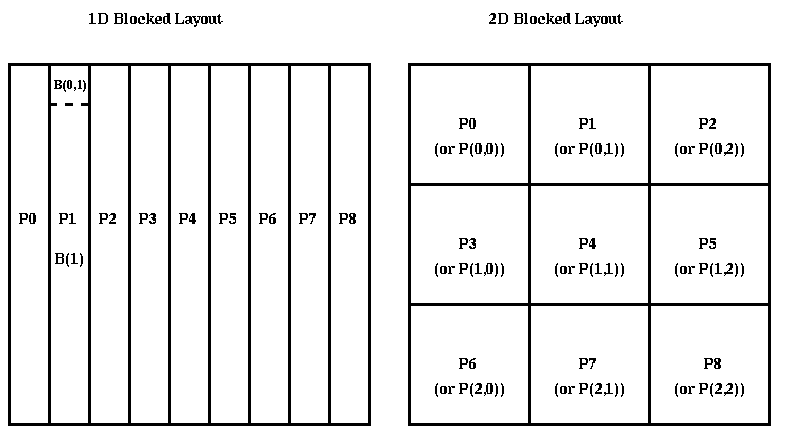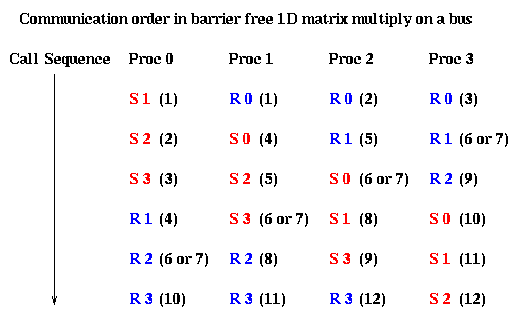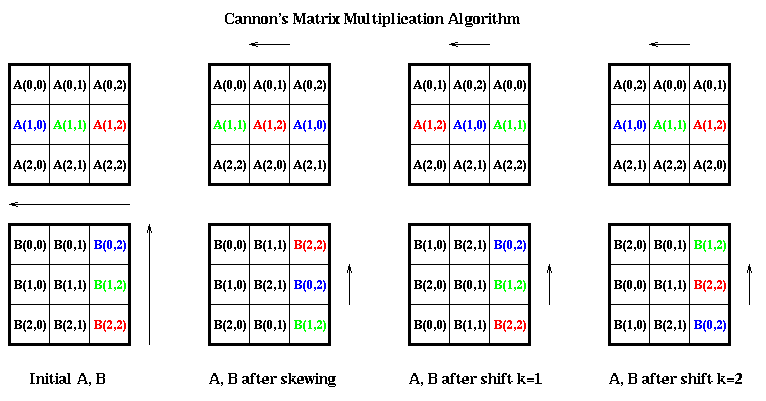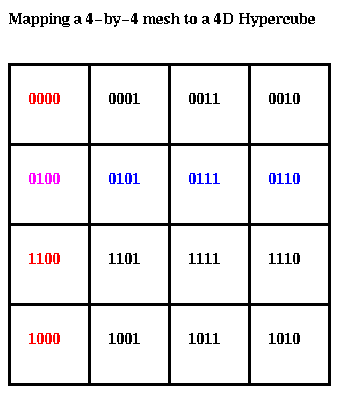# Parallel Matrix Multiplication

• Introduction
• 1D Blocked Layout on a Bus without Broadcast
• 1D Blocked Layout on a Bus with Broadcast
• 1D Blocked Layout on a Ring
• Cannon's algorithm on a 2D Mesh
• Matrix Multiplication on a 3D Mesh
• Matrix Multiplication on a Hypercube
• Gravity on a Hypercube
• Practical Parallel Software
• ## Introduction

We will show how to implement matrix multiplication C=C+A*B on several of the communication networks discussed in the last lecture, and develop performance models to predict how long they take. We will see that the performance depends on several factors.

• The interconnection network. Networks with higher connectivity, like the hypercube and mesh, permit faster algorithms than networks like rings and busses.
• The data layout, or where A, B and C are stored on the processors. The two most basic layouts are 1D blocked and 2D blocked, as illustrated below.
• The algorithm. We will use the standard algorithm requiring 2*n^3 arithmetic operations, so that the optimal parallel time on p processors will be 2*n^3/p time steps (arithmetic operations). Scheduling these operations will be the interesting part of the algorithm design.
•We will begin by examining the 1D Blocked Layout on a bus without broadcast (like a single ethernet), a bus with broadcast, and a ring of processors.

## 1D Blocked Layout on a Bus without Broadcast

For simplicity we will assume that n is divisible by p. As illustrated in the above figure, we will let B(i) denote the n-by-(n/p) part of matrix B owned by processor i, where i runs from 0 to p-1. A(i) and C(i) are analogous. We will also let B(j,i) denote the (n/p)-by-(n/p) submatrix of B, which is the submatrix of B(i) lying in rows j*(n/p) through (j+1)*(n/p)-1. For example, B(0,1) is shown in the above figure.

The algorithm depends on the following simple formula from linear algebra:

```      C(i) = C(i) + A*B(i) = C(i) + sum_{j=0}^{p-1} A(j)*B(j,i)
```
Since processor i owns C(i) and B(i), but not each A(j) as required by the formula, the algorithm will have to send each A(j) to each processor. This is similar to the Sharks and Fish 2 problem, fish moving under gravity, where each fish (A(j)) has to visit each processor in order to perform the computation.

Our first model of a bus is that at most one processor may send, and at most one may receive at a time. A floating point operation costs 1 time unit, message startup costs alpha time units, and the cost per work is beta time units. In other words, sending a message of n words takes alpha + beta*n time units. We use synchronous send and receive.

Here is the algorithm:

```  Matrix multiplication with a 1D blocked layout on a bus
without broadcast, and with a barrier.

C(MYPROC) = C(MYPROC) + A(MYPROC)*B(MYPROC,MYPROC)
for i=0 to p-1
for j=0 to p-1 except i
if ( MYPROC = i ) send A(i) to processor j
if ( MYPROC = j )
C(MYPROC) = C(MYPROC) + A(i)*B(i,MYPROC)
endif
barrier()
end for
end for
```
The cost of the arithmetic in the inner loop is 2*n*(n/p)^2 = 2*n^3/p^2, and the cost of the communication in the inner loop is alpha + n*(n/p)*beta, so the overall time is
```   Time = (p*(p-1)+1)*(2*n^3/p^2)             ... arithmetic
+ (p*(p-1))*( alpha + n*(n/p)*beta) ... communication

~ 2*n^3 + p^2*alpha + p*n^2*beta
```
where we have ignored lower order terms in p in the second formula. This is more than the serial time, 2*n^3, and grows with p rather than shrinking with p, and so is a rather poor parallel algorithm! In fact, it is not really parallel at all, since at most processor can compute at a time, because of the barrier.

So we can hope to do better by removing the barrier, in order to overlap computation and communication, and to send A(i) to several processors so they can operate in parallel. Here is the algorithm without a barrier:

```  Matrix multiplication with a 1D blocked layout on a bus
without broadcast, and without a barrier.

C(MYPROC) = C(MYPROC) + A(MYPROC)*B(MYPROC,MYPROC)
for i=0 to MYPROC-1
C(MYPROC) = C(MYPROC) + A(i)*B(i,MYPROC)
end for
for i=0 to p-1 except MYPROC
send A(MYPROC) to processor i
end for
for i=MYPROC+1 to p-1
C(MYPROC) = C(MYPROC) + A(i)*B(i,MYPROC)
end for
```
This program is non-deterministic, in the sense that the sends and receives in the algorithm without a barrier do not necessarily have to occur in the same order as the algorithm with a barrier. For example, the following figure shows the sequence in which sends and receives occur when there are 4 processors. There is one column for each processor, containing the sends and receives in the order each processor calls them; Sj means "send to proc j" and Ri means "receive from proc i". Each send and receive is labeled by the order in which it occurs. For example, "S2 (5)" in the column for processor 1 and "R1 (5)" in the column for processor 2 means that the fifth communication involves processor 1 sending to processor 2. Note that the two communications from processor 1 to 3, and from processor 2 to 0, may occur in either order (either may occur sixth or seventh). (Technically, we say the partial orders imposed on the communication events by the p programs on each processor do not constitute a total order.) This race condition is not important, because the same results are computed no matter what the order is.Let us analyze the performance of this algorithm. Intuitively, if the cost of a communication step,

```     cm = alpha + n*(n/p)*beta,
```
is sufficiently smaller than the cost of arithmetic in the inner loop,
```     ar = 2*n^3/p^2,
```
then efficiency should be high. Conversely, if communication is comparable to, or dominates, computation, we expect low efficiency. If we keep p fixed and let n grow, then since ar grows like n^3 and cm like n^2, we expect ar to dominate cm for large enough problems, and high efficiency to be achieved.

Now we will quantify this intuition. Let us draw the time-line for this algorithm, assuming that cm <= ar. In the time-line, a block of time labeled i->j refers to communication from processor i to j and takes time cm, and a block of time labeled jC refers to processor j computing, and takes time ar. For simplicity we assume communications occur in the same order as the program with a barrier, although as stated above this should not matter.

```  time --->
|   0C   |
|   1C   |
...
|   p-1C |
| 0->1 |   1C   |
| 0->2 |   2C   |
| 0->3 |   3C   |
...
|0->p-1| p-1C   |
...
```
As shown in the diagram, the overall computation forms a pipeline. To compute the duration of the pipeline, we need to consider whether there are any "bubbles" in the pipeline, i.e. if the communication steps cannot happen without intervening delays. In particular, the next communication to follow 0->p-1 is 1->0. 1->0 will be able to start without delay after 0->p-1 completes provided processor 1 is not busy, i.e. provided computation 1C has completed. This will be the case if ar <= (p-2)*cm, in which case the total time is
```    Time = p*(p-1)*cm + 2*ar
```
Using the inequality ar <= (p-2)*cm and some algebra yields
```    2*n^3/p = p*ar < Time <= (p^2+p-4)*cm
```
The lower bound 2*n^3/p is (nearly) attained when cm equals its lower bound ar/(p-2). This corresponds to (nearly) perfect speedup, since the serial time is 2*n^3. Thus, if communication is so fast that cm ~ ar/(p-2), the fact that the bus is a serial bottleneck does not matter. If cm is even smaller than ar/(p-2), so there are "bubbles" in the pipeline, the speedup can improve a little, but not much.

As cm grows, the speedup shrinks. When cm=ar, the running time is (p^2-p+2)*ar ~ 2*n^3, or approximately equal to the serial running time. In other words, parallelism does not help at all. If cm is larger than ar, the running time is slower than the serial algorithm. These possibilities are captured in the efficiency formula

```      Efficiency = Serial_Time / ( p * Parallel_Time )
= 1 / ( 2/p + (p-1)*cm/ar )
= 1 / ( 2/p + (p^3-p^2)/(2*n^3)*alpha
+ (p^2-p)/(2*n)*beta )
```
and we are assuming 1/(p-2) <= cm/ar <= 1. When cm/ar is close to 1/(p-2), efficiency is near 1. When cm/ar is close to 1, efficiency is nearly 1/p, i.e. there is no speedup at all. From the expression for n/p, we see that cm/ar is small when n>>p, and when alpha and beta are not too large. One could easily plug in particular values of n, p, alpha and beta to measure the efficiency for a particular matrix size on a particular machine.

## 1D Blocked Layout on a Bus with Broadcast

It is natural to ask how much the ability for many processors to receive a single message on the bus improves the performance of the above algorithm.

Here is the algorithm:

```  Matrix multiplication with a 1D blocked layout on a bus

C(MYPROC) = C(MYPROC) + A(MYPROC)*B(MYPROC,MYPROC)
for i=0 to p-1
if ( MYPROC = i )
else
endif
C(MYPROC) = C(MYPROC) + A(i)*B(i,MYPROC)
end for
```
Assuming the same communication model, the time is now
```   Time =  p*2*n^3/p^2 + p*( alpha + n^2/p*beta )
= 2*n^3/p + p*alpha + n^2*beta
```
so
```      Efficiency = Serial_Time / ( p * Parallel_Time )
= 1 / ( 1 + cm/ar )
= 1 / ( 1 +
p^2/(2*n^3)*alpha +
p/(2*n)*beta )
```
In contrast to the bus without broadcast, the cm/ar term in the denominator of the efficiency is p-1 times smaller, and so efficiency is a much less sensitive function of p. As before, since we expect alpha>>1 and beta>>1, we require n>>p for parallelism to be effective. However, since there is p times less communication time, our lower bound on n/p for effective parallelism is much lower than for a bus without broadcast, quantifying our intuition that more communication helps parallelism.

## 1D Blocked Layout on a Ring

It turns out that a ring of processors is adequate to get the performance just described; broadcasting is not necessary. The idea is similar to Sharks and Fish 2: we will use a ring to pass around the submatrices A(i). For simplicity, we assume each processor can send and receive simultaneously; at worst this underestimates the communication time by a factor of 2, since we can have odd numbered processors send to even numbered processors, and then even to odd.
```  Matrix multiplication with a 1D blocked layout on a ring

copy A(MYPROC) into T
C(MYPROC) = C(MYPROC) + T*B(MYPROC,MYPROC)
for i=1 to p-1
send T to processor MYPROC+1 mod p
receive T from processor MYPROC-1 mod p
C(MYPROC) = C(MYPROC) + T * B( (MYPROC-i) mod p, MYPROC )
end for
```
Assuming the same communication model, the time is now
```   Time = p*2*n^3/p^2 + (p-1)*( alpha + n^2/p*beta )
= 2*n^3/p + (p-1)*alpha + (p-1)/p * n^2*beta
```
and the efficiency is
```   Efficiency = 1 / ( 1 + (p-1)/p * cm/ar )
= 1 / ( 1 +
p*(p-1)/(2*n^3)*alpha +
(p-1)/(2*n)*beta )
```
which is just slightly faster than the time for the broadcast bus.

It is easy to see that this is essentially optimal, for a 1D blocked layout on a bus or ring, because the matrix multiplication formula we use requires every product A(i)*B(i,j) to eventually accumulate on processor j, which entails some data of size n*(n/p) moving from each processor i to each processor j, and this requires at least p-1 messages of size n^2/p.

Since a ring may be embedded in a mesh or hypercube, the same algorithm also works on these networks. But as we will see, much better algorithms are available.

## Cannon's algorithm on a 2D Mesh

First we describe Cannon's matrix multiplication algorithm for 2D blocked matrices without reference to the network, and later add the network. We assume that p is a perfect square p=s^2, and that n is divisible by s=sqrt(p). We let B(i,j) refer to the submatrix stored in processor P(i,j) in the above figure (note that this B(i,j) is not the same B(i,j) as in the algorithms for the 1D blocked layout!).

Cannon's algorithm reorders the summation in the inner loop of block matrix multiplication as follows:

```   C(i,j) = C(i,j) + sum_{k=0}^{s-1} A(i,k)*B(k,j)
= C(i,j) + sum_{k=0}^{s-1} A(i, (i+j+k) mod s)*B( (i+j+k) mod s, j)
```
```   Cannon's matrix multiplication algorithm

for all (i=0 to s-1)      ...  "skew" A
Left-circular-shift row i of A by i,
so that A(i,j) is overwritten by A(i, (j+i) mod s)
end for
for all (i=0 to s-1)      ... "skew" B
Up-circular-shift column i of B by i,
so that B(i,j) is overwritten by B( (i+j) mod s, j)

end for
for k=0 to s-1
for all (i=0 to s-1, j=0 to s-1)
C(i,j) = C(i,j) + A(i,j)*B(i,j)
Left-circular-shift each row of A by 1,
so that A(i,j) is overwritten by A(i, (j+1) mod s)
Up-circular-shift each column of B by 1,
so that B(i,j) is overwritten by B( (i+1) mod s, j)
end for
end for
```
The following picture illustrates the algorithm for 9 processors:In other words, after the initial "skewing", each data movement A(i,k) and B(k,j) arrive at processor P(i,j), and are multiplied and accumulated into C(i,j). The k's occur in different permutations on different processors, as described by (i+j+k) mod s.

For example, in the picture above the 3 colored blocks are accumulated to form C(1,2). First the blue blocks A(1,0) and B(0,2) are co-resident in P(1,2) and multiplied and accumulated. Then the green blocks A(1,1) and B(1,2) are multiplied and accumulated, and finally the red blocks A(1,2) and B(2,2) are multiplied and accumulated.

This algorithm is well suited to an s-by-s mesh of processors, for which we will now measure the performance.

• Skewing A. Each row of A can proceed independently of the others. By communicating with nearest neighbors, sending the message by the shortest path (left or right), and as before assuming a processor can send and receive simultaneously, the cost is
```         (s/2)*(alpha + (n/s)^2*beta) = sqrt(p)*alpha/2 + n^2/(2*sqrt(p))*beta
```
• Skewing B. The cost is the same as for skewing A.
• Shifting A (or B) left (or up) by 1. This costs alpha+n^2/p*beta.
• Local accumulation of A times B into C. This costs 2*(n/s)^3 = 2*n^3/p^(3/2).
• The total cost is therefore
```    Time = 2*n^3/p + 3*sqrt(p)*alpha + 3*n^2/sqrt(p) * beta
```
with efficiency
```    Efficiency = 1 / ( 1 +
1.5*p^(1.5)/n^3*alpha +
1.5*p^(.5)/n*beta )
```
Comparing with the time for a ring, we see the arithmetic time is the same, but the communication time is about sqrt(p) times smaller. This reflects the fact that the bisection width of a mesh is sqrt(p) times as large as a ring.

## Matrix Multiplication on a 3D Mesh

It is possible to decrease the cost of communication by another factor of p^(1/6) by using a 3D mesh instead of a 2D mesh as in the last section. The idea is to let the multiplication A(i,k)*B(k,j) in the formula
```   C(i,j) = C(i,j) + sum_{k=0}^{s-1} A(i,k)*B(k,j)
```
be done by processor (i,j,k) in the 3D mesh. Then the sums are accumulated along rows of the mesh. See "A three-dimensional approach to parallel matrix multiplication"by R. Agarwal et al, IBM J. of Res. and Dev., v. 39, n. 5, pp 521-600, Sept. 1995. for details.

## Matrix Multiplication on a Hypercube

Since a ring can be embedded in a hypercube, the same algorithm can be used there. But it turns out there is something even better.

We will first show how to map Cannon's algorithm to a hypercube, and then how to speed it up. Recall from the last lecture how we map a 2D mesh to a hypercube. Suppose the mesh is 2^m-by-2^m, where 2^m = s. Let G(m) = { g(0), ... , g(2^m-1) } be the m-bit Gray code. Then processor (i,j) in the mesh is mapped to processor g(i)*2^m+g(j) in the hypercube. In other words, we just concatenate the m bits in g(i) and the m bits in g(j) to get the hypercube address. An example for m=2 is shown below. Students of digital design will recognize that this is the same idea as a Karnaugh map.This mapping makes it clear that columns of the matrix are mapped to independent sub-hypercubes of the original hypercube, and the same for columns. For example, the blue column above is mapped into the blue sub-hypercube below, and the same for the red row and red sub-hypercube.Thus, we can use the more numerous wires of the hypercube to accelerate the skewing phase of Cannon's algorithm. This variation is due to Dekel, Nassimi and Sahni. We assume without loss of generality that A(i,j) is stored on processor i*2^m + j.

```  Dekel, Nassimi and Sahni's hypercube matrix multiply

for k= 0 to m-1
jk = 2^k and j  ... "logical and" of 2^k and j
ik = 2^k and i  ... "logical and" of 2^k and j
for all (i=0 to s-1, j=0 to s-1)
swap A(i, j xor ik) and A(i, j)   ... "exclusive or" of j and jk
swap B(jk xor i, j) and B(i, j)   ... "exclusive or" of jk and i
end for
for k=0 to s-1
for all (i=0 to s-1, j=0 to s-1)
C(i,j) = C(i,j) + A(i,j)*B(i,j)
Left-circular-shift each row of A by 1, in Gray code order
Up-circular-shift each column of B by 1, in Gray code order
end for
end for
```
The algorithm works as follows. After the skewing phase, A(i,j) has been moved to A(i,j xor i). This is accomplished by changing one bit of j at a time (from bit k=0 to bit k=m-1) to match the corresponding bit of j xor i. j xor ik can differ from j only in the k-th bit, so swapping with processor requires nearest neighbor communication only. Similarly, B(i,j) is moved to B(j xor i, j), one bit at a time. The cost of this skewing phase is 2*m*(alpha + (n/s)^2*beta), a factor of (sqrt(p)/2)/(2*m) = sqrt(p)/(2*log_2 p) times faster than Cannon on a mesh. The subsequent multiply-accumulate phase does not change in cost.

There is one more hypercube matrix-multiplication algorithm. To go faster than the last algorithm, it assumes that all log(p) wires connected to each processor can be used simultaneously, to get log(p) parallelism in communication; this corresponds to the hardware available on the CM-2. Rather than describe this algorithm, due to Ho, Johnsson and Edelman, in detail, we refer the reader to paper Parallel Numerical Linear Algebra, by Demmel, Heath, and van der Vorst, in volume 7 of the Class Reference Material. We reproduce the results below, for an n*2^n -by- n*2^n matrix on a 2*n-dimensional hypercube:

```      Algorithm       message startups   data sending steps   arithmetic steps
Cannon           2*(2^n-1)            2*n^2*(2^n-1)          2*n^3*2^n
Dekel et al      n+2^n-1              n^3 + n^2*(2^n-1)      2*n^3*2^n
Ho et al         n+2^n-1              n^3 + n*(2^n-1)        2*n^3*2^n
```
The final algorithm uses "all the wires all the time" on a hypercube, and is optimal in this sense. It was used in the matrix-multiplication routine in the CMSSL library on the CM-2.

### Gravity on a Hypercube

As mentioned above, matrix multiplication with a 1D layout on a ring of processors can be done using a communication pattern very similar to the one needed for computing gravity in the "Sharks and Fish 2 problem. We showed in Lecture 9 that a ring can be embedded in a hypercube, so this simple algorithm for gravity could still be used on a hypercube. But we can go much faster using the extra wires available to us in hypercubes.

In fact it is possible to embed d = log_2 p "nonoverlapping" rings into a d-dimensional hypercube, and use them all at once to get d times the bandwidth of a single ring. The CM-2 could in fact use all d wires at each node simultaneously. These rings actually do use the same wires, but do so at different times, and so are nonoverlapping in this sense.

If there are n fish, assign n/p to each processor, and further divide these into d groups of m=n/(p*d) fish. Each group F1,...,Fd of m fish will follow a different path through the node on the algorithm. The first path will be defined by the Gray Code G(d) defined above, the second path will consist of left_circular_shift_by_1(G(d)), i.e. each address in the Gray code will have its bits left shifted circularly by 1; this clearly maintains the Gray code property of having adjacent bit patterns differ in just 1 bit. The i-th path will be left_circular_shift_by_i(G(d)). The student is invited to draw a picture of this communication pattern, which uses "all-the-wires-all-the-time", an interesting feature of the CM-2 hypercube network.

## Practical Parallel Software

For portable parallel software for matrix multiplication and other Basic Linear Algebra Subroutines, see the PBLAS, or Parallel Basic Linear Algebra Subroutines. For a description of their design, see "A Proposal for a Set of Parallel Basic Linear Algebra Subprograms", J. Choi, J. Dongarra, S. Ostrouchov, A. Petitet, D. Walker, and R. C. Whaley, LAPACK Working Note 100, University of Tennessee Report CS-95-292, May 1995. More detailed algorithmic descriptions of these algorithms can be found in "PUMMA: Parallel Universal Matrix Multiplication Algorithms on Distributed Memory Concurrent Computers," by Jaeyoung Choi, Jack J. Dongarra, and David W. Walker LAPACK Working Note 57, University of Tennessee Report CS-93-187, May 1993, "SUMMA: Scalable Universal Matrix Multiplication Algorithm," by R. A. van de Geijn and J. Watts, LAPACK Working Note 96, University of Tennessee Report CS-95-286, April 1995, and "A User's Guide to the BLACS v1.0," by J. Dongarra and R. C. Whaley, LAPACK Working Note 94, University of Tennessee Report CS-95-286, April 1995.

We will return to describe this software, and higher level algorithms that use it, in a later lecture.